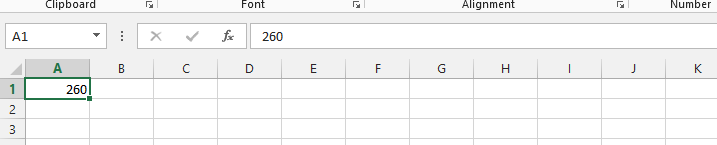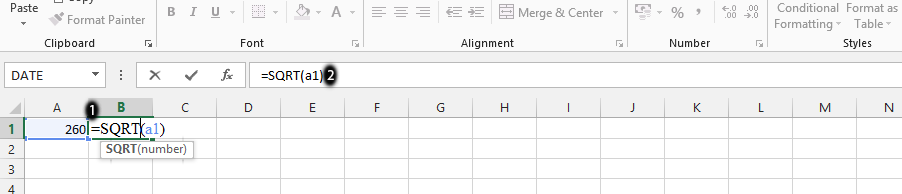#### How to calculate Square Root in Excel

Do you want to calculate the square root of a number in Excel? Here’s how:

## Square Root calculations

Enter the number that you want to find the square root of in a cell.In the next cell, type the formula =SQRT(cell_with_number).

Formula in the picture below is =SQRT(A1)The square root of the number appear in the cell.

## Understanding Square Roots in Excel

To calculate square roots in Excel, it’s crucial to grasp these principles:

Numeric Requirement: Ensure your input is numeric; text or formulas won’t work.

Avoid Negatives: Excel returns a #NUM! error when you try to find the square root of a negative number.

Decimal Outcomes: Square roots of decimals may not be integers, e.g., √1.44 = 1.2.

Custom Roots: For non-square roots, use “^” (e.g., √16 as 16^(1/2)) or the nth root calculator (e.g., ∛8 as 8^(1/3)).